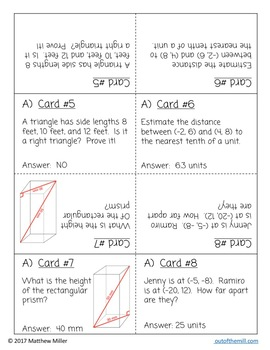# Speed Dating 8th Grade BundleSubject
Resource Type
File Type

PDF

(5 MB|54 pages)
Product Rating
4.0
(4 Ratings)
Standards
• Product Description
• StandardsNEW
Your students will be smiling and laughing as they practice 8th grade concepts with these fun Speed Dating activities. They won't even realize they are working on rigorous common core math standards!

This is a collection of five Math Speed Dating activities for 8th grade. Eighth grade standards such as exponent rules, Pythagorean Theorem, and scientific notation are included along with integer operations, a critical skill from seventh grade which students must understand to be successful with 8th grade standards such as solving equations and systems.

Included in this Product:
Integer Addition and Subtraction Speed Dating (7.NS.1)
This is a great activity to use to teach Speed Dating to your students, as it should be review from seventh grade.

Integer Operations Speed Dating (7.NS.1-2)
This builds on the previous activity, mixing together addition, subtraction, multiplication, and division with positive and negative numbers.

8.EE.1 Properties of Exponents: A Speed Dating Activity
Students practice the multiplication rule, division rule, and power of a power rule of exponents. They will also practice simplifying negative exponents.

8.EE.3 Scientific Notation Speed Dating
Students convert scientific notation to standard form and vice versa.

8.G.6-8 Pythagorean Theorem Speed Dating
Skills include determining if a triangle is a right triangle (8.G.6), finding missing right triangle side lengths and solving word problems (8.G.7), and finding the distance between two coordinates (8.G.8).

Also included are detailed instructions for the teacher, recording sheets for each activity, and sample filled out recording sheets.

Other activities you may like...
8.G.7 Pythagorean Theorem Scavenger Hunt
8.EE.3 Scientific Notation Cooperative Learning Activities
Slope and Unit Rate Cooperative Learning Bundle
Use numbers expressed in the form of a single digit times an integer power of 10 to estimate very large or very small quantities, and to express how many times as much one is than the other. For example, estimate the population of the United States as 3 × 10⁸ and the population of the world as 7 × 10⁹, and determine that the world population is more than 20 times larger.
Know and apply the properties of integer exponents to generate equivalent numerical expressions. For example, 3² × (3⁻⁵) = (3⁻³) = 1/3³ = 1/27.
Apply and extend previous understandings of multiplication and division and of fractions to multiply and divide rational numbers.
Apply and extend previous understandings of addition and subtraction to add and subtract rational numbers; represent addition and subtraction on a horizontal or vertical number line diagram.
Apply the Pythagorean Theorem to find the distance between two points in a coordinate system.
Total Pages
54 pages
Included
Teaching Duration
1 Week
Report this Resource to TpT
Reported resources will be reviewed by our team. Report this resource to let us know if this resource violates TpT’s content guidelines.